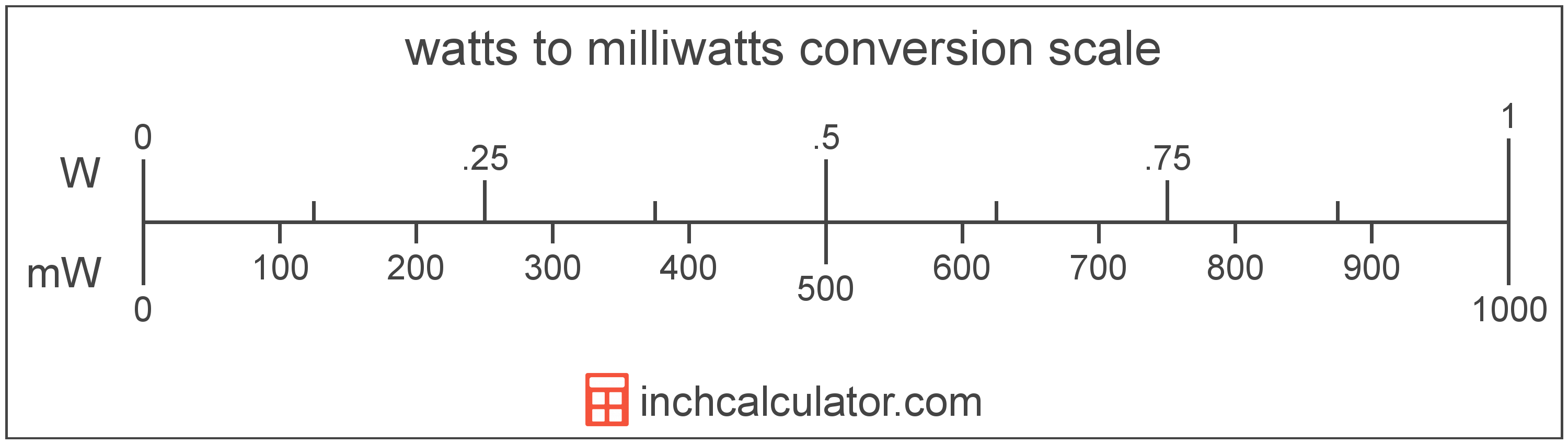# Watts to Milliwatts Conversion

Enter the power in watts below to get the value converted to milliwatts.

Results in Milliwatts:1,000 W = 1,000,000 mW
Do you want to convert milliwatts to watts?

## How to Convert Watts to MilliwattsTo convert a watt measurement to a milliwatt measurement, multiply the power by the conversion ratio.

Since one watt is equal to 1,000 milliwatts, you can use this simple formula to convert:

milliwatts = watts × 1,000

The power in milliwatts is equal to the watts multiplied by 1,000.

For example, here's how to convert 5 watts to milliwatts using the formula above.
5 W = (5 × 1,000) = 5,000 mW

### How Many Milliwatts are in a Watt?

There are 1,000 milliwatts in a watt, which is why we use this value in the formula above.

1 W = 1,000 mW

## Watts

One watt is the power equal to the rate of energy of one joule per second in an electrical circuit. One watt is also equal to the power produced in a circuit with a voltage potential of one volt with a current of one amp.

The watt is the SI derived unit for power in the metric system. Watts can be abbreviated as W; for example, 1 watt can be written as 1 W.

Watt's Law states the relationship between power, current, and voltage. Using Watt's Law, it's possible to express the power in watts as an expression using current and voltage.

PW = IA × VV

The power in watts is equal to the current in amperes times the potential difference in volts.

Combining this with Ohm's Law, it's also possible to express the power in watts using resistance in addition to volts and amps.

## Milliwatts

One milliwatt is the power equal to 1/1,000 of a watt, or the energy consumption at a rate of 1/1,000 of a joule per second.

The milliwatt is a multiple of the watt, which is the SI derived unit for power. In the metric system, "milli" is the prefix for 10-3. Milliwatts can be abbreviated as mW; for example, 1 milliwatt can be written as 1 mW.

## Watt to Milliwatt Conversion Table

Watt measurements converted to milliwatts
Watts Milliwatts
0.001 W 1 mW
0.002 W 2 mW
0.003 W 3 mW
0.004 W 4 mW
0.005 W 5 mW
0.006 W 6 mW
0.007 W 7 mW
0.008 W 8 mW
0.009 W 9 mW
0.01 W 10 mW
0.02 W 20 mW
0.03 W 30 mW
0.04 W 40 mW
0.05 W 50 mW
0.06 W 60 mW
0.07 W 70 mW
0.08 W 80 mW
0.09 W 90 mW
0.1 W 100 mW
0.2 W 200 mW
0.3 W 300 mW
0.4 W 400 mW
0.5 W 500 mW
0.6 W 600 mW
0.7 W 700 mW
0.8 W 800 mW
0.9 W 900 mW
1 W 1,000 mW

## References

1. International Bureau of Weights and Measures, The International System of Units, 9th Edition, 2019, https://www.bipm.org/documents/20126/41483022/SI-Brochure-9-EN.pdf